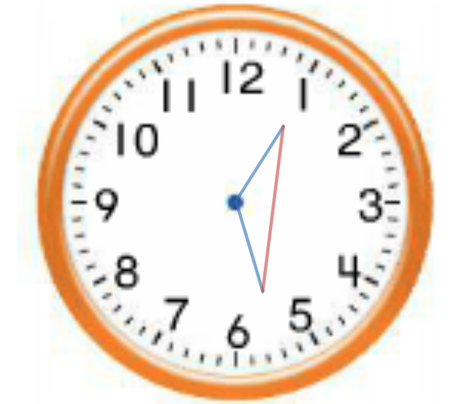# Clock Fantasyjackal_1586Cefalo SUST Inter Univers...154/167/297
Limits 2s, 512 MB

One day the author imagined an analog clock with minute and hour arms. The thought was simple: if we consider the two arms as two sides of a triangle and join the separate ends of the arms for the third side of the triangle, then at different times throughout the day and night, the area of the triangle varies. A natural question then arises, given the lengths of minute and hour arms, what is the maximum area of the triangle?In this picture, the blue line segments represent minute and hour arms, and the red line segment represents the third side of the triangle.

## Input

The first line of the input represents the number of test cases $T ( 0 < T < 500001)$. The following $T$ lines each contain two floating point numbers $a$ and $b (0 < a, b \leq 10^8)$ representing the length of the hour and the minute arms, respectively.

## Output

For each case, print the maximum area that can be achieved at some position of the hour and minute arms as described above. Errors less than $10^{-6}$ will be ignored.

## Sample

InputOutput
1
661.5611881304 354.5250382063

117270.002748868### Submit